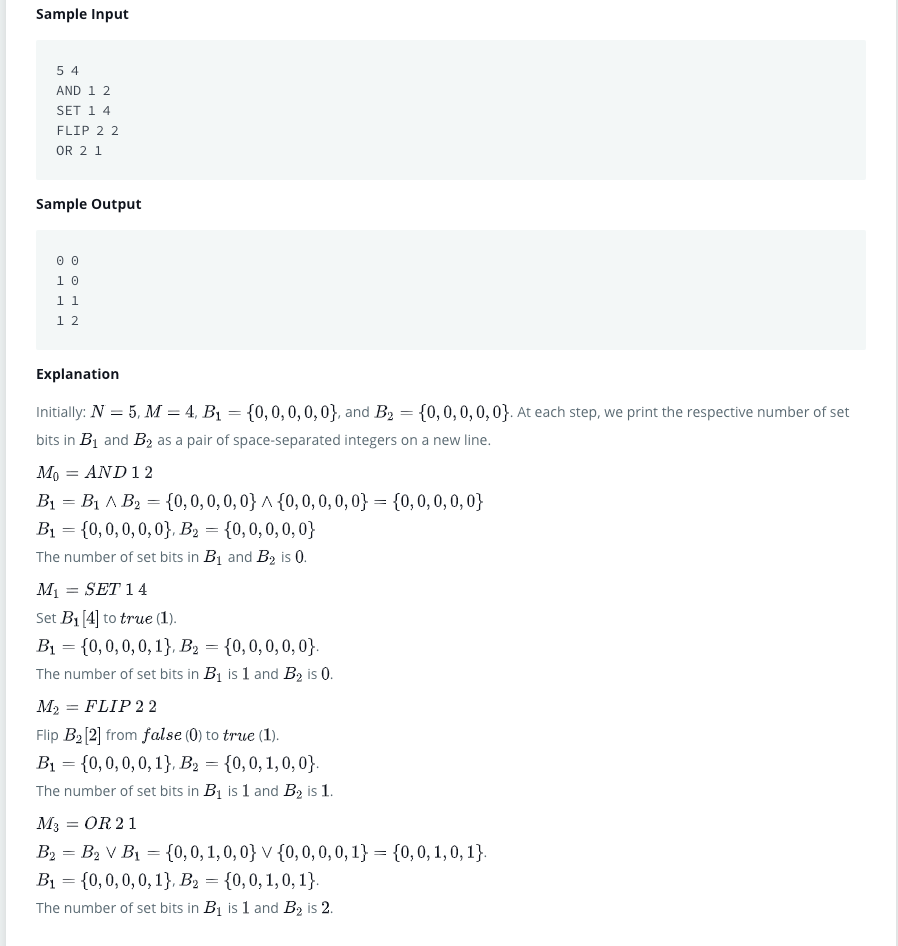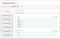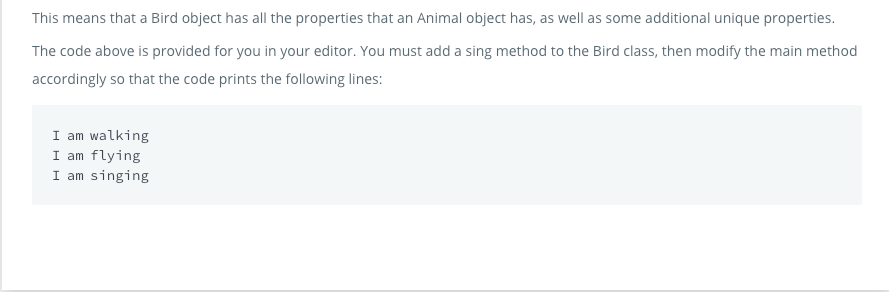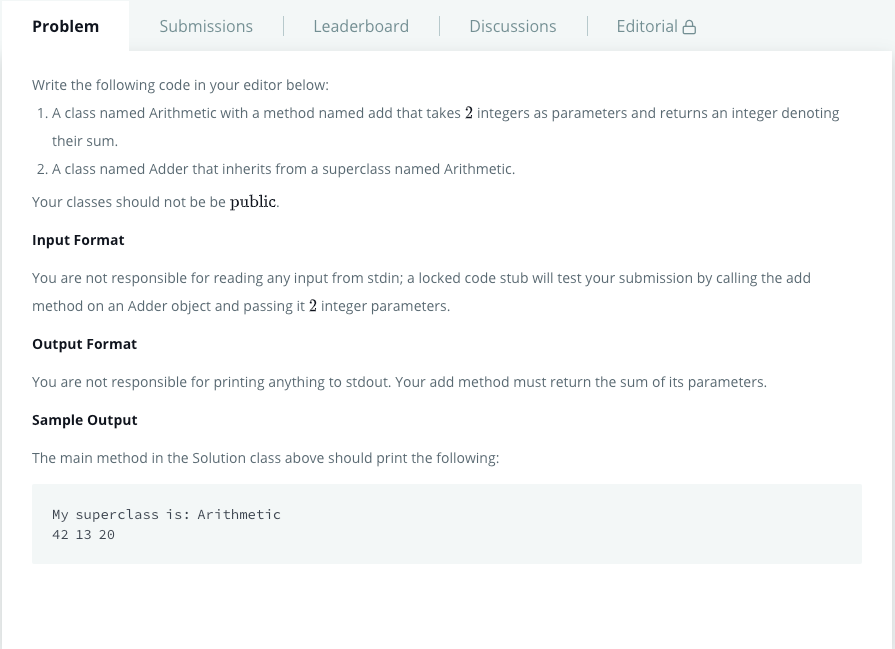# 07/31/2020Photo by Liana Mikah on Unsplash

## Java BitSetJava BitSet, retrieved from https://www.hackerrank.com/challenges/java-bitset/problem
`import java.io.*;import java.util.*;public class Solution {    public static void main(String[] args) {        /* Enter your code here. Read input from STDIN. Print output to STDOUT. Your class should be named Solution. */        Scanner input = new Scanner(System.in);        int size = input.nextInt();        int op = input.nextInt();        BitSet bs1 = new BitSet(a);        BitSet bs2 = new BitSet(b);        while (op -- > 0){            String s = input.nextLine();            int x = input.nextInt();            int y = input.nextInt();            if (s.equals("AND")) {                if (x == 1) {                    bs1.and(bs2);                }                else {                    bs2.and(bs1);                }            }            else if ( s.equals("OR")) {                if (x == 1) {                    bs1.or(bs2);                }                else {                    bs2.or(bs1);                }            }            else if ( s.equals("XOR")) {                if (x == 1) {                    bs1.xor(bs2);                }                else {                    bs2.xor(bs1);                }            }            else if (s.equals("FLIP")) {                if (x == 1){                    bs1.flip(y);                }                else {                    bs2.flip(y);                }            }            else {                if (x == 1){                    bs1.set(y);                }                else {                    bs2.set(y);                }            }        }        System.out.println(bs1.cardinality() + " " + bs2.cardinality());    }}`Runtime Error
`import java.io.*;import java.util.*;public class Solution {    public static void main(String[] args) {        /* Enter your code here. Read input from STDIN. Print output to STDOUT. Your class should be named Solution. */        Scanner input = new Scanner(System.in);        int size = input.nextInt();        int op = input.nextInt();        BitSet bs1 = new BitSet(size);        BitSet bs2 = new BitSet(size);import java.io.*;import java.util.*;        public class Solution {            public static void main(String[] args) {                /* Enter your code here. Read input from STDIN. Print output to STDOUT. Your class should be named Solution. */                Scanner input = new Scanner(System.in);                int size = input.nextInt();                int op = input.nextInt();                BitSet bs1 = new BitSet(size);                BitSet bs2 = new BitSet(size);                while (op -- > 0) {                    String s = input.nextLine();                    int i = input.nextInt();                    int j = input.nextInt();                    switch (s) {                        case("AND"):                            if (i == 1) {                                bs1.and(bs2);                            } else {                                bs2.and(bs1);                            }                        case("OR"):                            if (i == 1) {                                bs1.or(bs2);                            }                            else {                                bs2.or(bs1);                            }                        case("XOR"):                            if (i == 1) {                                bs1.xor(bs2);                            }                            else{                                bs2.xor(bs1);                            }                        case("FLIP"):                            if (i == 1) {                                bs1.flip(j);                            }                            else {                                bs2.flip(j);                            }                        case("SET"):                            if (i == 1){                                bs1.set(j);                            }                            else {                                bs2.set(j);                            }                    }                }                System.out.println(bs1.cardinality() + " " + bs2.cardinality());            }        }        while (op -- > 0) {            String s = input.nextLine();            int i = input.nextInt();            int j = input.nextInt();            switch (s) {                case("AND"):                    if (i == 1) {                        bs1.and(bs2);                    } else {                        bs2.and(bs1);                    }                case("OR"):                    if (i == 1) {                        bs1.or(bs2);                    }                    else {                        bs2.or(bs1);                    }                case("XOR"):                    if (i == 1) {                        bs1.xor(bs2);                    }                    else{                        bs2.xor(bs1);                    }                case("FLIP"):                    if (i == 1) {                        bs1.flip(j);                    }                    else {                        bs2.flip(j);                    }                case("SET"):                    if (i == 1){                        bs1.set(j);                    }                    else {                        bs2.set(j);                    }            }        }        System.out.println(bs1.cardinality() + " " + bs2.cardinality());    }}`
`import java.io.*;import java.util.*;public class Solution {    public static void main(String[] args) {        /* Enter your code here. Read input from STDIN. Print output to STDOUT. Your class should be named Solution. */        Scanner input = new Scanner(System.in);        int size = input.nextInt();        int op = input.nextInt();        BitSet bs1 = new BitSet(size);        BitSet bs2 = new BitSet(size);        while (op -- > 0) {            String s = input.next();            int i = input.nextInt();            int j = input.nextInt();            switch (s) {                case("AND"):                    if (i == 1) {                        bs1.and(bs2);                    } else {                        bs2.and(bs1);                    }                    break;                case("OR"):                    if (i == 1) {                        bs1.or(bs2);                    }                    else {                        bs2.or(bs1);                    }                    break;                case("XOR"):                    if (i == 1) {                        bs1.xor(bs2);                    }                    else{                        bs2.xor(bs1);                    }                    break;                case("FLIP"):                    if (i == 1) {                        bs1.flip(j);                    }                    else {                        bs2.flip(j);                    }                    break;                case("SET"):                    if (i == 1){                        bs1.set(j);                    }                    else {                        bs2.set(j);                    }                    break;            }            System.out.println(bs1.cardinality() + " " + bs2.cardinality());        }        input.close();    }}`

## Java Inheritance IJava Inheritance I, retrieved from https://www.hackerrank.com/challenges/java-inheritance-1/problem
`import java.io.*;import java.util.*;import java.text.*;import java.math.*;import java.util.regex.*;class Animal{    void walk()    {        System.out.println("I am walking");    }}class Bird extends Animal{    void fly()    {        System.out.println("I am flying");    }    void sing() {        System.out.println("I am singing");    }}public class Solution{    public static void main(String args[]){        Bird bird = new Bird();        bird.walk();        bird.fly();        bird.sing();    }}`

## Java Inheritance IIJava Inheritance II, retrieved from https://www.hackerrank.com/challenges/java-inheritance-2/problem
`import java.io.*;import java.util.*;import java.text.*;import java.math.*;import java.util.regex.*;//Write your code hereclass Arithmetic {    public int calc(int a, int b) {        int sum = a + b;        return sum;    }}class Adder extends Arithmetic {    public int add(int x, int y) {        return calc(x, y);    }}public class Solution{    public static void main(String []args){        // Create a new Adder object        Adder a = new Adder();        // Print the name of the superclass on a new line        System.out.println("My superclass is: " + a.getClass().getSuperclass().getName());        // Print the result of 3 calls to Adder's `add(int,int)` method as 3 space-separated integers:        System.out.print(a.add(10,32) + " " + a.add(10,3) + " " + a.add(10,10) + "\n");    }}`

## References:

Undergraduate student / Research assistant/ Always curious / Opinions are mine